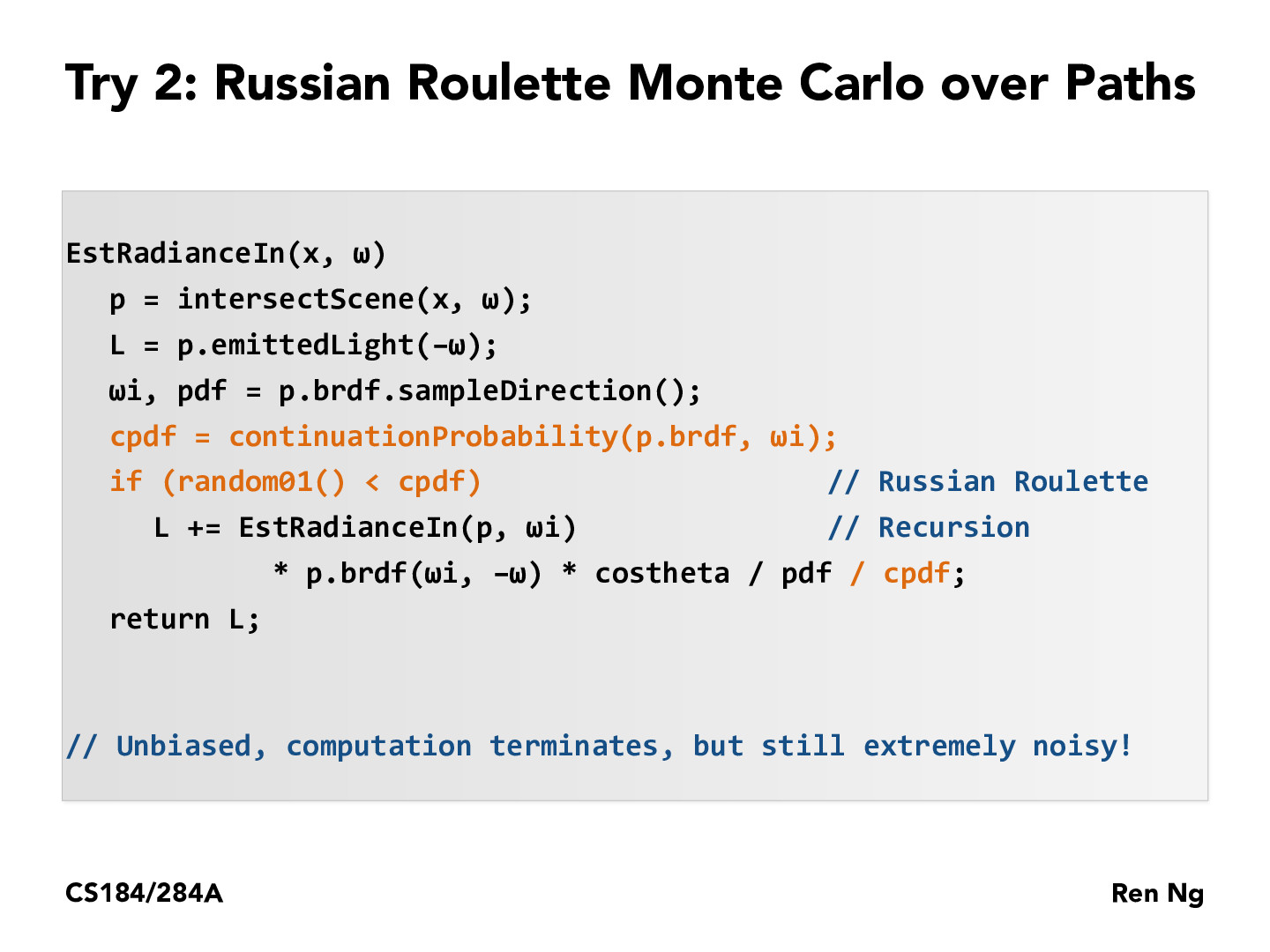Lecture 13: Global Illumination & Path Tracing (83)rileylyman184

I'm not sure how this is the Monte Carlo estimator for the radiance into point x on the screen. We are setting L to be L_e at first. So so far we have that L_o = L_e part of the equation down. The full equation is L_o = L_e + int(brdf * L_i *cos). So now we need to estimate that integral. But we are only taking one estimate of that integral at angle w_i when we do L+= EstRadianceIn(p, w_i) + ... . Why are we not doing this in a for-loop for some number of times to get a good estimate of the integral??elizabethyli

I think this pseudocode function is supposed to be in the same context as the other ones presented earlier in lecture, where the function is essentially just one iteration. To actually do the monte-carlo estimator, you would have to call this function inside a loop until L stops changing.ziyaointl

@elizabethyli I think you are right that we need to call this function in a for loop. However, I think the number of samples N for Monte Carlo is usually predefined instead of waiting for the average to converge, which could take a long time.

Correction: methods that wait for a pixel to converge seem to be more effective than using one N for everything. See part 5 of assignment 3-1.

You must be enrolled in the course to comment